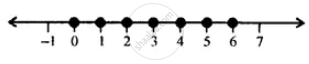# Solve and Graph the Solution Set on a Number Line: 2/3"X" + 5 <= 1/2"X" + 6; "X" ∈ "W" - Mathematics

Sum

Solve and graph the solution set on a number line: 2/3"x" + 5 <= 1/2"x" + 6; "x" ∈ "W"

#### Solution

2/3"x" + 5 <= 1/2"x" + 6

=> 2/3"x" xx 6 + 5 xx 6 <= 1/2 "x" xx 6 + 6 xx 6   ....(Multiplying both sides by 6)

⇒ 4x + 30 ≤ 3x + 36

⇒ 4x - 3x + 30 ≤ 3x - 3x + 36  ...(Substracting 3x from both sides)

⇒ x + 30 ≤ 36

⇒ x + 30 - 30 ≤ 36 - 30    ...(Substracting 30 from both sides)

x ≤ 6

∴ The required graph is:Concept: Operation on of Whole Number on Number Line
Is there an error in this question or solution?

#### APPEARS IN

Selina Concise Mathematics Class 8 ICSE
Chapter 15 Linear Inequations
Exercise 15 (B) | Q 10 | Page 175